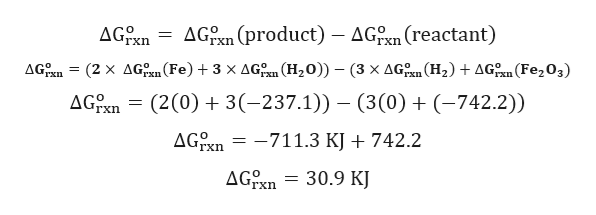# Using any data you can find in the ALEKS Data resource, calculate the quilibrium constant K at 25.0°C for the following reaction. Fe2O3(s)+  3H2(g)→  2Fe(s)+  3H2O(l)Round your answer to 2 significant digits. delta G of Fe2O3=-742.2 kj/molh2=0Fe=0H20=-237.1kj/mol

Question
744 views

Using any data you can find in the ALEKS Data resource, calculate the quilibrium constant K at 25.0°C for the following reaction.

Fe2O3(s)+  3H2(g)→  2Fe(s)+  3H2O(l)

delta G of Fe2O3=-742.2 kj/mol

h2=0

Fe=0

H20=-237.1kj/mol

check_circle

Step 1

Given chemical reaction:

Fe2O3(s) +  3H2(g)  2Fe(s)+  3H2O(l)

Step 2

Calculation for ΔGo ...help_outlineImage TranscriptioncloseAGn AGPn (product) - AGn (reactant) 'rxn AGEn = (2 x AG(Fe)3 x AG (H20)) - (3 x AGn (H2)AG (Fe203) Ixn AG On = (2(0) 3(-237.1)) - (3 (0)(-742.2)) AGTxn -711.3 KJ742.2 AGn 30.9 KJ гxn fullscreen

### Want to see the full answer?

See Solution

#### Want to see this answer and more?

Solutions are written by subject experts who are available 24/7. Questions are typically answered within 1 hour.*

See Solution
*Response times may vary by subject and question.
Tagged in

### Chemistry